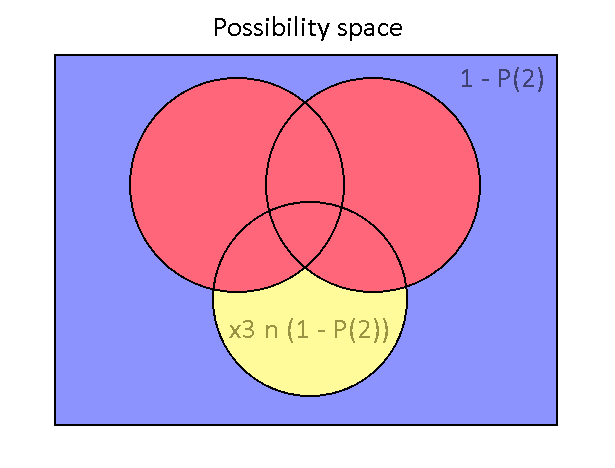# Recursive Formulas of Sums of Dependent Events

Time to talk about sets! Specifically, sums of probabilities of dependent events.

Let’s use the deck of cards as the classic example for statistics problems. What is the probability of finding a card that is a club? There are 4 suits with the same number of cards for each suit, so the chance is ¼. What about the probability of finding a card that is a 7? Well, there are 13 possible values (2-10, Jack, Queen, King, Ace) for a card to have, each having an equal chance of appearing, so that would be 1/13.

Chance of a club: ¼

Chance of a 7: 1/13

What is the chance of finding a club AND a 7? We need to multiply the event probabilities together since they are independent events. So, (¼)(1/13) which is (1/52). This makes sense since there is only one 7 of clubs in the 52 card deck. If we need to find the probability of event A AND event B, then that is represented by an intersection (A n B)

What is the chance of finding a club OR a diamond? Since no cards can be both a club or a diamond, we can simply add the probabilities together, so (¼) + (¼) = ½.

What about the chance of finding a club OR a 7? The addition is less simple, since if we add up all cards that are clubs with all cards that are 7’s, then we counted the card that is a 7 of clubs twice. So, we have to subtract that intersection out. This would give us (¼) + (1/13) - (¼)(1/13) = 16/52 chance. 13 cards are clubs, 4 cards are 7’s, and we subtract by the over-counted 7 of clubs to get our total of 13 + 4 - 1 = 16 cards. This union formula for two dependent events A and B is this:

``` A u B = A + B - (A n B) ```

Here’s a diagram explaining this all graphically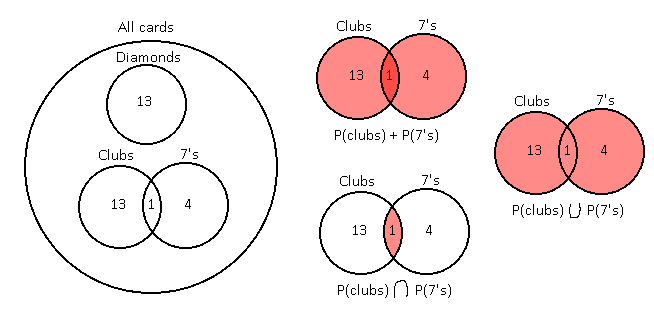The goal of summing probabilities of dependent events is to make sure that the intersecting circles are counted only once. For two overlapping circles that formula is this:

``` A u B = A + B - (A n B) ```

There exists formulas for more than two circles, and they start to get ugly really quickly. Let’s work out how to find out the sums of 3 dependent events. If we simply sum all three probabilities (shade all circles) then we get lots of overlapping, with darker spots meaning more overlapping.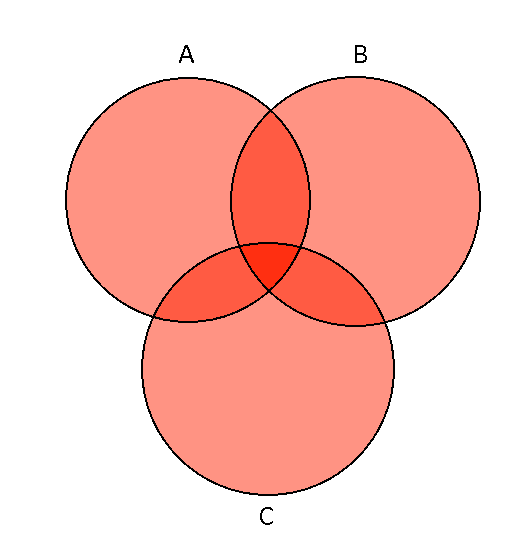A + B + C is a good start, but all of the intersections need to be removed. We want every space to be filled just once. So, subtract (A n B), (A n C), and (B n C) to get this: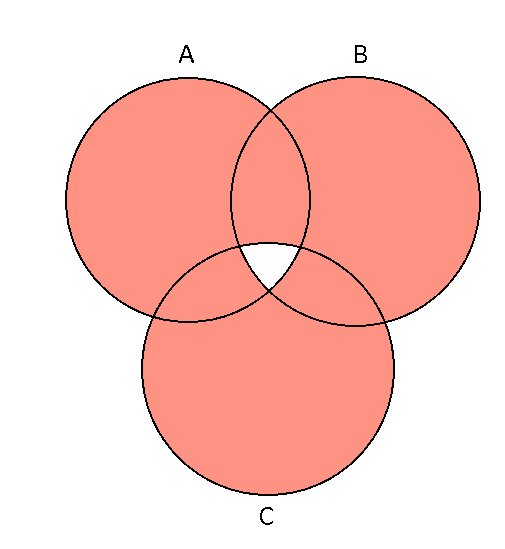The intersecting shapes have now been counted 1 time instead of 2 times, but the very center shape was counted only 3 times before. This area intersects with all other shapes, so we subtracted that area 3 times in total, thus not counting it at all. The final step is to include that center shape again through (A n B n C).

So, this is the final equation:

``` A + B + C - (A n B) - (B n C) - (A n C) + (A n B n C). ```

I’m going to introduce a shorter notation, where (A n B) = AB. This is not multiplication. It would be if were talking about independent events, but we are not. Just keep that in mind. Anyways, here are our two formulas so far, one for two events and one for three events.

``` A u B = A + B - AB ```

``` A u B u C = A + B + C - AB - BC - AC + ABC ```

Here is a challenge for you: try and find the formula for 4 events. You may notice some interesting patterns while doing this, and it will be a great setup for the next cool thing I’m about to show. Check below for the answer.

``` A u B u C u D = A + B + C + D - AB - AC - AD - BC - BD - CD + ABC + ABD + BCD - ABCD ```

One thing to notice is that each grouping of events with the same number of events being grouped all have a consistent sign: they are either all positive or all negative. Another even more interesting fact is that all of the terms that exist in a 3-event sum exist in any higher-event sums. So, for a 4-event sum, all the terms in a 2-event sum and a 3-event sum exist in that 4-event sum. This means that we can use the power of recursion to define higher-event sums through definitions of lower-event sums!

I will now change the syntax to something more flexible. All unique events are specified as x1, x2, x3, x4, etc. representing event 1, event 2, event 3, event 4, and so on. Here is one last piece of syntactic sugar. When I mean (x1 u x2) I will now mean P(2), meaning the probability of 2 dependent events summed up. Here are the first two formulas again:

``` P(2) = x1 + x2 - x1x2 ```

``` P(3) = x1 + x2 + x3 - x1x2 - x2x3 - x1x3 + x1x2x3 ```

Substitute the terms that make up a 2-event sum with P(2) to get this:

``` P(3) = P(2) + x3 - x2x3 - x1x3 + x1x2x3 ```

We can take this reduction one step farther by noticing that all the left over terms have a ‘factor’ of x3 (again, this isn’t multiplication. We are just abusing some multiplicative properties here)

``` P(3) = P(2) + x3(1 - x2 - x1 + x1x2) ```

(x3 n 1) would just evaluate to x3, since 1 is a 100% probability chance. We can reduce this even FURTHER by looking at the terms inside the parentheses. The terms inside there are the same as the terms in the 2-event sum formula! Just, all the terms’ signs are flipped. So, flip them.

``` P(2) + x3(1 - x2 - x1 + x1x2) = P(2) + x3(1 - (x1 + x2 - x1x2)) = P(2) + x3(1 - P(2)) ```

That’s a really nice equation. Turns out we can do the same thing with the definition of P(2). Technically, P(1) is just x1, so knowing that, P(2) can be reduced to a similar form through 'factoring’.

```            ```
P(1) = x1
P(2) = x1 + x2 - x1x2 = P(1) + x2 - x1x2 = P(1) + x2(1 - x1) = P(1) + x2(1 - P(1))
P(2) = P(1) + x2(1 - P(1))
P(3) = P(2) + x3(1 - P(2))

```
```

This pattern continues for P(4). The proof is simple and is left as an exercise for the reader. Anyways, this pattern allows us to recursively define the formula for any P(N) as this:

```            ```
P(1) = x1
P(N) = P(N-1) + xN(1 - P(N-1))

```
```

This is cool, but this can be taken a step further by visualizing what this means graphically for our circles. Imagine we had a two-circle graph correctly colored in and we wanted to figure out what the three-circle graph version would look like. Based on this recursive formula, we can start with the two-circle graph and build up to the three-circle graph (and any graph with more circles!). Here’s the setup.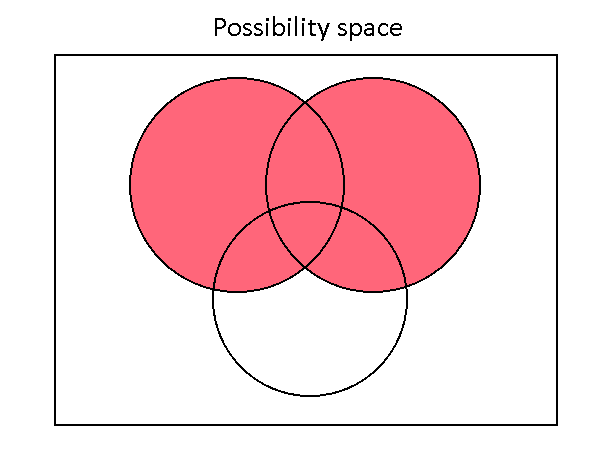The rectangle around everything represents all possibilities of these events. So, a completely filled in rectangle means a 100% probability. Currently (A u B) is filled in, and we want to color in just the white parts inside the third circle below without over-coloring. Let’s look back at the formula.

``` P(3) = P(2) + x3(1 - P(2)) ```

P(2) is figured out for us, but what about this x3(1 - P(2)) term? Remember that this is equivalent to x3 n (1 - P(2)). What is 1 - P(2)? It is like coloring the whole box, and then subtracting out the colors in the two circles. So, 1 - P(2) is actually all of the remaining whitespace inside the box! So, that area is intersected with x3, the third circle. What this means is that that x3(1 - P(2)) is the intersection between all of the whitespace and the third circle. This actually leaves us with exactly the white space inside the third circle, and nothing more, thus successfully filling in the 3-circle graph correctly.